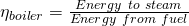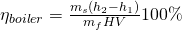Sim Labs

# 1 Boiler Efficiency

### Learning Objectives

Operate the Plant in the following modes and compute the Boiler Thermal Efficiency

• 230 MW Burning HFO
• 80% Load Burning Bio Fuel
• 80% Load Burning Coal with soot built up.

# Theory

First we define efficiency. There are many ways to present it, for example, efficiency is the ability to do something without wasting energy, effort or time. Put it mathematically, it is the ratio of useful output to thermal energy input. Layperson often uses the terms efficiency and effectiveness interchangeably however efficiency is to do with minimizing waste and effectiveness to do with maximizing output (more on this in Heat Exchangers Lab). Boiler efficiency is sometimes defined as combustion efficiency which is computed by the ratio of the burner’s capability to burn fuel completely to the unburnt fuel and excess air in the exhaust. Thermal efficiency on the other hand, indicates the heat exchanger’s (i.e. boiler’s) capacity to transfer heat from the combustion process to the water or steam in the boiler. In general, the maximum boiler efficiency attainable from a boiler depends on such factors as method of burning the fuel, design of the furnace and heat transfer surfaces. In addition, the type of fuel, boiler load and operational practices influence the boiler efficiency. In this lab, we focus on the fuel type, boiler load and best practices.

## Boiler Thermal Efficiency

Next we express the Boiler Thermal Efficiency as follows:Where energy to steam is the heat transfer required to form steam. Let,

h2=specific enthalpy of steam formed, [kJ/kg],

h1=specific enthalpy of feed water, [kJ/kg].

Because the steam is formed at constant pressure, heat transfer required to form 1 kg of steam in the boiler is

Energy to Steam= (h2-h1) [kJ]

Energy from fuel is computed from the mass of fuel used and its heat (calorific) value. For coal this is the heating value as measured in a bomb calorimeter and it corresponds to the internal energy of combustion. If

mf= mass of fuel burned in a given time

ms= mass of steam generated in the same time

HV= heat value of fuel [kJ/kg]

Energy from fuel= mf x HV [kJ]

Then we can write:### Lab Instructions

You will run 4 different initial conditions in this lab:

• I10 230 MW Burning HFO
• I15 80% Load Burning Bio Fuel
• I14 80% Load Burning Coal
• I14 80% Load Burning Coal and use MD250 to set up soot variables.

For each condition collect the relevant data to compute the Boiler Thermal Efficiency.

## Hints & Tips

For data collection, use trends as shown below:

In addition to various pressure, temperature and flow values, you will need to log the following tags in your trends:

• H00810 HFO heat value
• H00870 Pellet heat value (bio fuel)
• H00830 Coal heat value

To calculate the enthalpy values, you may use an app or online tool such as the Superheated Steam Table: https://goo.gl/GdVM4U

For the coal operation with soot built up, use MD250 and set malfunctions as follows:

### Deliverables

Your lab report is to include the following:

• Trend plots: Supply all plots taken for each of the 4 conditions,
• Computation: Use MATLAB or MS Excel and calculate the boiler thermal efficiency for the 4 conditions specified,
• Conclusion: Write a summary (max. 500 words, in a text box if using Excel) comparing your results and suggestions for further study.